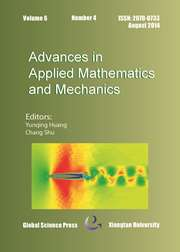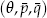Home
Hostname: page-component-7ccbd9845f-dzwm5 Total loading time: 0.79 Render date: 2023-01-29T02:57:19.284Z Has data issue: true Feature Flags: { "useRatesEcommerce": false } hasContentIssue trueAdvances in Applied Mathematics and Mechanics

# Nonlinear Stability and B-convergence of Additive Runge-Kutta Methods for Nonlinear Stiff Problems

Published online by Cambridge University Press:  29 May 2015

*
*Corresponding author. Email: xag@xtu.edu.cn (A. G. Xiao)

## Abstract

In this paper, we are devoted to nonlinear stability and B-convergence of additive Runge-Kutta (ARK) methods for nonlinear stiff problems with multiple stiffness. The concept of-algebraic stability of ARK methods for a class of stiff problems Kστ is introduced, and it is proven that this stability implies some contractive properties of the ARK methods. Some results on optimal B-convergence of ARK methods for Kσ,0 are given. These new results extend the existing ones of RK methods and ARK methods in the references. Numerical examples test the correctness of our theoretical analysis.

Type
Research Article
Information
Advances in Applied Mathematics and Mechanics , August 2015 , pp. 472 - 495

## Access options

Get access to the full version of this content by using one of the access options below. (Log in options will check for institutional or personal access. Content may require purchase if you do not have access.)

## References

Araújo, A., A note on B-stability of splitting methods, Computing Visual. Sci., 6 (2004), pp. 5357.CrossRefGoogle Scholar
Araújo, A., Murua, A. and Sanz-Serna, J. M., Symplectic methods based on decompositions, SIAM J. Numer. Anal., 34(5) (1997), pp. 19261947.CrossRefGoogle Scholar
Ascher, U. M., Ruuth, S. J. and Spiteri, R. J., Implicit-explicit Runge-Kutta methods for time-dependent partal differential equations, Appl. Numer. Math., 25 (1997), pp. 151167.CrossRefGoogle Scholar
Boscarino, S. and Russo, G., Flux-explicit IMEX Runge-Kutta schemes for hyperbolic to parabolic relaxation problems, SIAM J. Numer. Anal., 51(1) (2013), pp. 163190.CrossRefGoogle Scholar
Bujanda, B. and Jorge, J. C., Stability results for fractional step discretization of time dependent coefficient evolutionary problems, Appl. Numer. Math., 38 (2001), pp. 6985.CrossRefGoogle Scholar
Bujanda, B. and Jorge, J. C., Additive Runge-Kutta methods for the resolution of linear parabolic problems, J. Comput. Appl. Math., 140 (2002), pp. 99117.CrossRefGoogle Scholar
Bujanda, B. and Jorge, J. C., Efficient linear implicit methods for nonlinear multidimensional parabolic problems, J. Comput. Appl. Math., 164165 (2004), pp. 159174.Google Scholar
Burrage, K., Hundsdorfer, J. C. and Verwer, J. G., A study of B-convergence of Runge-Kutta methods, Computing, 36 (1986), pp. 1734.CrossRefGoogle Scholar
Burrage, K. and Hundsdorfer, W. H., The order of B-convergence of algebraically stable Runge-Kutta methods, Bit, 27 (1987), pp. 6271.CrossRefGoogle Scholar
Burrage, K. and Butcher, J. C., Stability criteria for implicit Runge-Kutta methods, SIAM J. Numer. Anal., 16(1) (1979), pp. 4657.CrossRefGoogle Scholar
Dekker, K. and Verwer, J. G., Stability of Runge-Kutta Methods for Stiff Nonlinear Differential Equations, North-Holland, Amsterdam, 1984.Google Scholar
Donat, R., Higueras, I. and Martinez-Gavara, A., Some theoretical results about stability for IMEX schemes applied to hyperbolic equations with stiff reaction terms, Kreiss, G.et al. (eds.), Numerical Mathematics and Advanced Applications, 2009, DOI 10.1007/978–3-642–11795-4 29, Springer-Verlag, 2010.Google Scholar
Frank, R., Schneid, J. and Ueberhuber, C. W., The concept of B-convergence, SIAM J. Numer. Anal., 18(5) (1981), pp. 753780.CrossRefGoogle Scholar
Frank, R., Schneid, J. and Ueberhuber, C. W., Stability properties of implicit Runge-Kutta methods, SIAM J. Numer. Anal., 22(3) (1985), pp. 497514.CrossRefGoogle Scholar
Frank, R., Schneid, J. and Ueberhuber, C. W., Order results of implicit Runge-Kutta methods applied stiff systems, SIAM J. Numer. Anal., 22(3) (1985), pp. 515534.CrossRefGoogle Scholar
Garćia-Celayeta, B., Higueras, I. and Roldán, T., Contractivity/ monotonicity for additive Runge-Kutta methods: Inner product norms, Appl. Numer. Math., 56 (2006), pp. 862878.CrossRefGoogle Scholar
Hairer, E. and Wanner, G., Solving Ordinary Differential Equations, Stiff and Differential-Algebraic Problems, Springer-Verlag, 1996.Google Scholar
Higueras, I., Strong stability of additive Runge-Kutta methods, SIAM J. Numer. Anal., 44(4) (2006), pp. 17351758.CrossRefGoogle Scholar
Higueras, I., Mantas, J. M. and Roldán, T., Design and implementation of predictors for additive semi-implicit Runge-Kutta methods, SIAM J. Sci. Comput., 31(3) (2009), pp. 21312150.CrossRefGoogle Scholar
Hundsdorfer, W. and Verwer, J., Numerical Solution of Time-Dependent Advection-Diffusion-Reaction Equations, Springer Series in Computational Mathematics, Springer, Berlin, 2003.CrossRefGoogle Scholar
Kennedy, C. A. and Carpenter, M. H., Additive Runge-Kutta schemes for convection-diffusion-reaction equations, Appl. Numer. Math., 44(1–2) (2003), pp. 139181.CrossRefGoogle Scholar
Koto, T., IMEX Runge-Kutta schemes for reaction-diffusion equations, J. Comput. Appl. Math., 215 (2008), pp. 182195.CrossRefGoogle Scholar
Kupka, F., Happenhofer, N., Higueras, I. and Koch, O., Total-variation-diminishing implicit-explicit Runge-Kutta methods for the simulation of double-diffusive convection in astrophysics, J. Comput. Phys., 231 (2012), pp. 3561–358.CrossRefGoogle Scholar
Liu, H. Y. and Zou, J., Some new additive Runge-Kutta methods and their applications, J. Comput. Appi. Math., 190(1–2) (2006), pp. 7498.CrossRefGoogle Scholar
Li, S. F., Theory of Computational Methods for Stiff Differential Equations, Hunan Science and Technology Press, 1997 (in Chinese).Google Scholar
Maeyama, S., Ishizawa, A., Watanabe, T. H., Nakajima, N., Tsuji-Iio, S. and T-Sutsui, H., A hybrid method of semi-Lagrangian and additive semi-implicit Runge-Kutta schemes for gyrokinetic Vlasov simulations, Computer Phys. Commun., 183 (2012), pp. 19861992.CrossRefGoogle Scholar
Pareschi, L. and Russo, G., Implicit-explicit Runge-Kutta schemes and applications to hyperbolic systems with relaxation, J. Sci. Comput., 25 (2005), pp. 129155.Google Scholar
Schlegel, M., Knolha, O., Arnold, M. and Wolke, R., Numerical solution of’multiscale problems in atmospheric modeling, Appl. Numer. Math., 62 (2012), pp. 15311543.CrossRefGoogle Scholar
Xiao, A. G., The order of B-convergence of Runge-Kutta methods, Natural Sci. J. Xiangtan University, 14(2) (1992), pp. 1619.Google Scholar
Xiao, A. G.,-algebraic stability of Runge-Kutta methods, Mathematica Numerica Sinica, 15(4) (1993), pp. 440448.Google Scholar
Zhong, X. L., Additive semi-implicit Runge-Kutta methods for computing high speed noneauilib-rium reactive flows, J. Comput. Phys., 128 (1996), pp. 1931.CrossRefGoogle Scholar
2
Cited by

# Save article to Kindle

Note you can select to save to either the @free.kindle.com or @kindle.com variations. ‘@free.kindle.com’ emails are free but can only be saved to your device when it is connected to wi-fi. ‘@kindle.com’ emails can be delivered even when you are not connected to wi-fi, but note that service fees apply.

Find out more about the Kindle Personal Document Service.

Nonlinear Stability and B-convergence of Additive Runge-Kutta Methods for Nonlinear Stiff Problems
Available formats
×

# Save article to Dropbox

To save this article to your Dropbox account, please select one or more formats and confirm that you agree to abide by our usage policies. If this is the first time you used this feature, you will be asked to authorise Cambridge Core to connect with your Dropbox account. Find out more about saving content to Dropbox.

Nonlinear Stability and B-convergence of Additive Runge-Kutta Methods for Nonlinear Stiff Problems
Available formats
×

# Save article to Google Drive

To save this article to your Google Drive account, please select one or more formats and confirm that you agree to abide by our usage policies. If this is the first time you used this feature, you will be asked to authorise Cambridge Core to connect with your Google Drive account. Find out more about saving content to Google Drive.

Nonlinear Stability and B-convergence of Additive Runge-Kutta Methods for Nonlinear Stiff Problems
Available formats
×
×